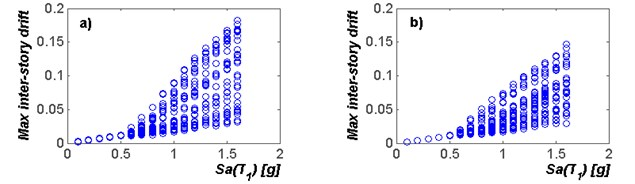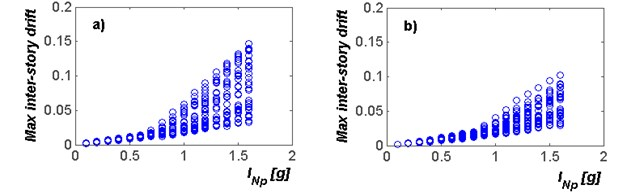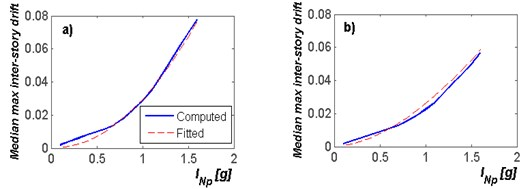Published: 30 May 2017

# Maximum inter-story drift demands of steel frames in terms of the intensity measure INp

Edén Bojórquez1
Victor Baca2
Juan Bojórquez3
Alfredo Reyes-Salazar4
Robespierre Chávez5
María Hernández6
1, 2, 3, 4, 5, 6Facultad de Ingeniería, Universidad Autónoma de Sinaloa, Calzada de las Américas y B. Universitarios s/n, C.P. 80040, Culiacán, Sinaloa, México
Corresponding Author:
Edén Bojórquez
Views 115

#### Abstract

In the present work, a new equation to predict the maximum inter-story drift demands of mid-rise steel framed buildings is proposed in terms of a new ground motion intensity measures based on the spectral shape. For this aim, the maxim\um inter-story drift of steel frames with 4, 6, 8 and 10 stories subjected to several narrow-band ground motions is estimated as a function of the spectral acceleration at first mode of vibration $Sa\left({T}_{1}\right)$, which is commonly used in earthquake engineering and seismology, and with a new parameter related to the structural response known as ${I}_{Np}$. It is observed that the spectral-shape-based intensity measure ${I}_{Np}$ is the parameter best related with the structural response of the selected steel frames under narrow-band motions. For this reason, an equation to compute the maximum inter-story drift demand of mid-rise steel frames as a function of ${I}_{Np}$ is proposed. The equation is useful for the rapid seismic assessment.

## 1. Introduction

The uncertainty of the structural response of buildings under several earthquake ground motions can be reduced if an efficient intensity measure (IM) is selected, where the efficiency is defined as the ability to predict the response of structures subjected to earthquakes with low uncertainty. Several studies have been developed with the aim to observe the relation between intensity measures and the seismic response of structures, and various intensity measures have been proposed [1-16]. Currently, several studies promote the use of vector-valued or scalar ground motion IMs based on spectral shape, because they predict with good accuracy the maximum inter-story drift and maximum ductility of structures subjected to earthquakes [8, 12-13]. In particular, vector and scalar ground motion intensity measures based on ${N}_{p}$ which are representative of the spectral shape have resulted very well related with the nonlinear structural response in terms of peak and energy demands [12-14, 17, 18]. Moreover, the parameter ${N}_{p}$ has been successfully used for ground motion record selection . Nevertheless, most of the studies to illustrate the potential of ${N}_{p}$-based intensity measures are related to the spectral shape in terms of acceleration were only the standard deviation of the maximum inter-story drift has been obtained, and it is necessary to estimate the relation between promising intensity measures and peak drift demands. In addition, it is important to establish equations to compute the structural demands in terms of ground motion intensity measures as Cornell and co-workers suggest . For this reason, the first objective of the present paper is to compare the efficiency of the new ground motion intensity measure ${I}_{Np}$ which is based on the spectral shape parameter ${N}_{p}$ in comparison with the most used intensity measure around the world which is the spectral acceleration at first mode of vibration. Then simplified expressions to compute the maximum inter-story drift demands of mid-rise steel framed buildings in terms of ${I}_{Np}$ are proposed.

## 2. Methodology

### 2.1. Definition of the selected intensity measures

In the last years, the most used ground motion intensity measure by earthquake engineers, seismologists, and seismic design guidelines is the spectral acceleration at first mode of vibration. This parameter is very useful because is the perfect predictor of seismic response of elastic single degree of freedom systems and it is a good option for predicting the response of elastic multi degree of freedom structures dominated by the first mode of vibration. In addition, studies have demonstrated the sufficiency of $Sa\left({T}_{1}\right)$ with respect to magnitude and distance [7, 21]. Recently, various studies have demonstrated the inefficiency of $Sa\left({T}_{1}\right)$, for example to predict the response of buildings under near source ground motion records and narrow-band motions [9, 12], energy demands [12, 22] and so on. The limitations of spectral acceleration at first mode of vibration can be clearly observed with the elastic response spectra where the scatter in the spectral shape due to the effect of the elongated period, or some spectral ordinates at higher mode periods is not considered. Inspired by this issues Bojórquez and Iervolino  have proposed the parameter ${N}_{p}$ and the ${I}_{Np}$ intensity measure. Although these parameters have been described in works developed by the first author and co-workers, in the next section with the aim to better understanding the potential of ${N}_{p}$ and ${I}_{Np}$, they are defined.

### 2.2. The spectral shape parameter ${\mathbit{N}}_{\mathbit{p}}$

Recent studies suggest that the spectral shape is crucial to predict the structural response of buildings under earthquakes and for this reason the earthquake engineering and seismology community has highlighted the limitations of spectral acceleration at first mode of vibration. For example, $Sa\left({T}_{1}\right)$ does not provide information about the spectral shape in other regions of the spectrum, which may be important for the nonlinear behavior (beyond ${T}_{1}$) or for structures dominated by higher modes (before ${T}_{1}$). In the case of nonlinear shaking, the structure may be sensitive to different spectral values associated to a range of periods defined, from the fundamental period and a limit value of practical interest, say ${T}_{N}$. This calls for intensity measures providing information about the spectral shape in a whole region of the spectrum as $〈Sa,{R}_{T1,T2}〉$ and $S{a}_{avg}\left({T}_{1},\dots ,{T}_{N}\right)$, where ${R}_{T1,T2}$ is defined as the ratio between the spectral acceleration at the period T2 divided by the spectral acceleration at period ${T}_{1}$ and $S{a}_{avg}\left({T}_{1},\dots ,{T}_{N}\right)$ is the geometrical mean between the period ${T}_{1}$ and ${T}_{N}$. Although parameters as$S{a}_{avg}\left({T}_{1},\dots ,{T}_{N}\right)$ or the area under the spectrum, account for the spectral shape, a specific value of $S{a}_{avg}\left({T}_{1},\dots ,{T}_{N}\right)$ or area under the spectrum may be associated with different patterns of the spectrum between ${T}_{1}$ and ${T}_{N}$, that is, with different spectral shapes. A useful improvement may be the use of $S{a}_{avg}\left({T}_{1},\dots ,{T}_{N}\right)$ normalized with respect to $Sa\left({T}_{1}\right)$. To this aim the spectral shape parameter named Np (Eq. 1) was proposed by Bojórquez and Iervolino :

1
${N}_{p}=\frac{S{a}_{avg}\left({T}_{1},\dots ,{T}_{N}\right)}{Sa\left({T}_{1}\right)}.$

### 2.3. ${\mathbit{I}}_{\mathbit{N}\mathbit{p}}$ ground motion intensity measure

To incorporate the effects of nonlinear behavior in the prediction of structural response, Bojórquez and Iervolino proposed a new scalar ground motion intensity measure based on $Sa\left({T}_{1}\right)$ and ${N}_{p}$ defined as:

2
${I}_{Np}=Sa\left({T}_{1}\right){{N}_{p}}^{\alpha }.$

In Eq. (2) the $\alpha$ value has to be determined from regression analysis. Analyses developed by Bojórquez and Iervolino and others [12, 17] suggest that the optimal values of $\alpha$are close to 0.4.

### 2.4. Steel structures

Four steel frames having 4, 6, 8 and 10 stories were considered for the study. The frames are denoted as F4, F6, F8 and F10, and they were designed according to the Mexico City Seismic Design Provisions having three eight-meter bays and story heights of 3.5 meters. A36 steel was used for the beams and columns of the frames. For the dynamic analysis of the steel frames models, the RUAUMOKO program  was used. Relevant characteristics for each frame, such as the fundamental period of vibration (${T}_{1}$), and the seismic coefficient (${C}_{y}$) are shown in Table 1 (the latter value was established from static nonlinear analyses). An elasto-plastic model with 3 % strain-hardening was used to represent the cyclic behavior of the transverse sections located at both ends of the steel beams and columns, and 3 % of critical damping was assigned to the first two modes of vibration of the frames.

Table 1Characteristics of the structural steel frame models

 Frame Number of stories Period of vibration (s) ${C}_{y}$ ${T}_{1}$ F4 4 0.90 0.45 F6 6 1.07 0.42 F8 8 1.20 0.38 F10 10 1.35 0.36

### 2.5. Earthquake ground motion records

A set of 30 narrow-banded ground motions recorded at Lake Zone sites of Mexico City was considered (see Fig. 1). All the motions were recorded at sites having soil periods near of two seconds, during seismic events with magnitudes near of seven or larger and having epicenters located at distances of 300 km or more from Mexico City. It should be mentioned that sites having soil periods of two seconds are fairly common within the Lake Zone and that the higher levels of shaking (in terms of peak ground acceleration PGA and velocity PGV) have been consistently observed at these sites. Moreover, most of the structural damages in the well-known 1985 Mexican earthquake occurred in the selected sites.

### 2.6. Incremental dynamic analysis

To observe the effectiveness of spectral acceleration and ${I}_{Np}$ to predict the maximum inter-story drift demands of the selected steel frames under narrow-band motions, incremental dynamic analysis is used . For this aim, the records are scaled at different spectral acceleration and ${I}_{Np}$ values. Fig. 3 illustrate a typical plot of incremental dynamic analysis results for $Sa\left({T}_{1}\right)$ in terms of maximum inter-story drift demands calculated for frame models F4 and F6 under the selected narrow-band motions. It is observed a clear relation among $Sa\left({T}_{1}\right)$ and drift demands; however, the uncertainty to predict peaks demands using the spectral acceleration tend to increase if the level of intensity of the earthquake ground motion increases. For example for $Sa\left({T}_{1}\right)$ values smaller than 0.5 g, spectral acceleration is an excellent candidate as intensity measure since the uncertainty in the prediction is despicable, because the seismic response of the steel structure is almost linear elastic. Nevertheless, for values of intensities equals to 1.5 g, the maximum inter-story drifts are in the range of 0.02 up to 0.125, which indicate large uncertainty and the limitations of $Sa\left({T}_{1}\right)$ to predict the seismic response of this structure for large levels of nonlinear behavior. Thus, it is necessary to use new intensity measures with better prediction capacity of the structural response as in the case of ${I}_{Np}$ as can be observed in Fig. 4. In this figure, incremental dynamic analysis for frames F4 and F6 is illustrated, where the horizontal axis corresponds to the records scaled at different ${I}_{Np}$ values, and the vertical to the maximum inter-story drift. It is observed that for small intensity values, ${I}_{Np}$ is an excellent predictor of the structural response. Moreover, for large values of intensities, the range of maximum inter-story drift demands at a specific level of ${I}_{Np}$ is not so large as in the case of $Sa\left({T}_{1}\right)$. For example, the range of peak drifts is from 0.05 until 0.1 for ${I}_{Np}$ values of 1.6 g, indicating the advantages of using the new intensity measure ${I}_{Np}$ in comparison with the spectral acceleration at first mode of vibration. The results suggest that large uncertainty is associated with the spectral acceleration as intensity measure. Thus, ${I}_{Np}$ can characterize with better efficiency the seismic response of buildings under narrow-band motions, at least for the selected cases of the present study. This motivates the objective of the present study which is to propose simplified equations to compute maximum inter-story drift demands of steel frames as a function of ${I}_{Np}$. Note that this study corresponds to the firsts efforts related to scale records for a specific ${I}_{Np}$ value.

Fig. 3Incremental dynamic analysis for the selected mid-rise steel frames under narrow-band motions using SaT1 as intensity measure: a) F4; b) F6Fig. 4Incremental dynamic analysis for the selected mid-rise steel frames under narrow-band motions using INp as intensity measure: a) F4; b) F6## 3. Equations to predict maximum inter-story drifts as a function of ${\mathbit{I}}_{\mathbit{N}\mathbit{p}}$

In this part of the present study, a simplified equation to estimate maximum inter-story drift demands of steel frames under narrow-band ground motions is proposed. The equation has the form suggested by Cornell et al. , which is well-known as the power law model. This equation indicates that given the level of intensity (for example spectral acceleration), the predicted median inter-story drift demand can be represented as follows:

3
$\stackrel{^}{\gamma }=a\left(IM{\right)}^{b},$

where $\stackrel{^}{\gamma }$ is the median value of the maximum inter-story drift demand; $IM$ is the selected intensity measure, in this study ${I}_{Np}$ is used as intensity measure; and finally, $a$ and $b$ are parameters that must be calibrated in the regression. In general, the equations to obtain the median value of the maximum inter-story drift in terms of ${I}_{Np}$ can be expressed as follows:

4
$\stackrel{^}{\gamma }=a\left({I}_{Np}{\right)}^{b}.$

Fig. 5 shows the results of the fitted developed for the Frame F4 and F6 using ${I}_{Np}$ as intensity measure. It is observed that the regression suggested a very well fitted with the median maximum inter-story drift values obtained from the incremental dynamic analyses. The values of $a$ and $b$ are expressed in terms of the ratio of structural period divided by the soil period $T/Ts$ (Eqs. (5-6)). Eqs. (5-6) are substituted in the above proposed simplified equation to predict the median maximum inter-story drift (Eq. (4)) as a function of the ground motion intensity measure ${I}_{Np}$ (see Eq. (7)). It is important to say that the proposed equation is very useful for a rapid estimation of the structural response of buildings:

5
$a=-0.0412\left(T/{T}_{s}\right)+0.048,$
6
$b=-3.6419\left(T/{T}_{s}\right)+3.677.$
7
$\stackrel{^}{\gamma }=\left(-0.041T/{T}_{s}+0.048\right){{I}_{Np}}^{-3.642T/{T}_{s}+3.677}.$

Fig. 5Median inter-story drift demands obtained from incremental dynamic analysis of the mid-rise steel frames in terms of INp for frames a) F4; b) F6## 4. Conclusions

In this study, the efficiency of spectral acceleration at first mode of vibration to predict the seismic response of mid-rise steel framed buildings under narrow-band motions was compared with a recently proposed ground motion intensity measure accounting for nonlinear behavior, which is known as ${I}_{Np}$. It was observed the advantages of ${I}_{Np}$ in comparison with $Sa\left({T}_{1}\right)$, where the uncertainty to predict the maximum inter-story drift demands of the selected steel models was considerably reduced. Furthermore, a simplified equation to predict the median value of the maximum inter-story drift demands of mid-rise frames subjected to narrow-band ground motions in terms of ${I}_{Np}$ has been proposed. Note that the equation is valid for steel framed buildings with structural vibration periods smaller than the soil period. The equations proposed are very useful for the rapid assessment of the structural damage, seismic fragility estimation, structural reliability, life cycle cost and so on.

31 March 2017
Accepted
12 April 2017
Published
30 May 2017
SUBJECTS
Seismic engineering and applications
Keywords
intensity measure
spectral shape
inter-story drifts
steel frames
Acknowledgements

The support given by El Consejo Nacional de Ciencia y Tecnología is appreciated. Financial support also was received from the Universidad Autónoma de Sinaloa under Grant PROFAPI.